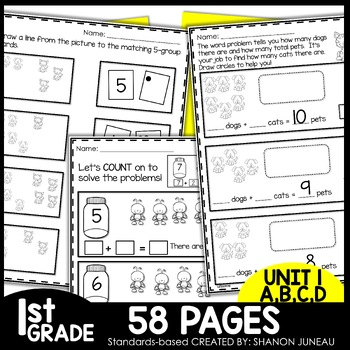Subject
Resource Type
File Type

PDF

(21 MB|25 pages)
Standards
Also included in:
1. These print and go math worksheets are perfect for math centers, independent practice, sub tubs, and assessments. This Math Bundle covers:missing addends simple word problems using pictures number bonds using 10, 9, 8, 7 as the whole, counting on, and finding the sumcommutative property of addition
\$12.25
\$9.80
Save \$2.45
2. These print and go NO PREP math worksheets are the perfect addition to your first grade curriculum. They cover all first grade concepts in an easy to read layout. These are perfect for reinforcement of skills taught, homework, independent work, or group work. These are GREAT for printable packets
\$63.50
\$47.62
Save \$15.88
• Product Description
• StandardsNEW

❤️If you are looking for a combo of printable and digital resource, you've found it! This is perfect to use during distance learning. These Print and Go NO PREP 1st Grade Math Worksheets cover:

• simple word problems using pictures
• number bonds using 10, 9, 8, 7 as the whole
• counting on
• finding the sum

These are perfect for extra practice, remediation, homework, and centers! If you use Eureka Math these are great first grade math worksheets that can be used as supplemental activities. These can also be used as a printable AND/or a DIGITAL pack for distance learning and can also be used as Independent Work Packets for early morning work or work from home packets.

PLEASE READ DIRECTIONS HERE. KEEP IN MIND THAT THIS IS IN BETA MODE WITH TPT. You can check out the VIDEO here!

BUNDLED FOR CONVENIENCE

If you are looking for other resources that go along with this lesson, see the items below:

CLICK HERE TO FOLLOW ME! Following me allows for you to see items that I put in my store for 1/2 price for the first 24 hours.

I SURE APPRECIATE YOU!

❤️Shanon Juneau - We Are Better Together❤️

Determine the unknown whole number in an addition or subtraction equation relating three whole numbers. For example, determine the unknown number that makes the equation true in each of the equations 8 + ? = 11, 5 = ▯ - 3, 6 + 6 = ▯.
Add and subtract within 20, demonstrating fluency for addition and subtraction within 10. Use strategies such as counting on; making ten (e.g., 8 + 6 = 8 + 2 + 4 = 10 + 4 = 14); decomposing a number leading to a ten (e.g., 13 - 4 = 13 - 3 - 1 = 10 - 1 = 9); using the relationship between addition and subtraction (e.g., knowing that 8 + 4 = 12, one knows 12 - 8 = 4); and creating equivalent but easier or known sums (e.g., adding 6 + 7 by creating the known equivalent 6 + 6 + 1 = 12 + 1 = 13).
Relate counting to addition and subtraction (e.g., by counting on 2 to add 2).
Use addition and subtraction within 20 to solve word problems involving situations of adding to, taking from, putting together, taking apart, and comparing, with unknowns in all positions, e.g., by using objects, drawings, and equations with a symbol for the unknown number to represent the problem.
Total Pages
25 pages
N/A
Teaching Duration
N/A
Report this Resource to TpT
Reported resources will be reviewed by our team. Report this resource to let us know if this resource violates TpT’s content guidelines.